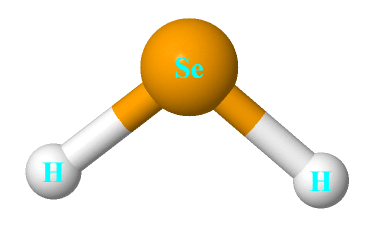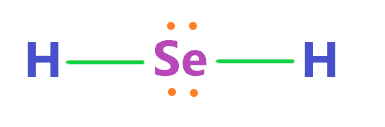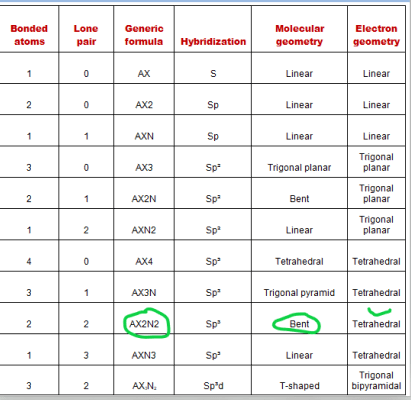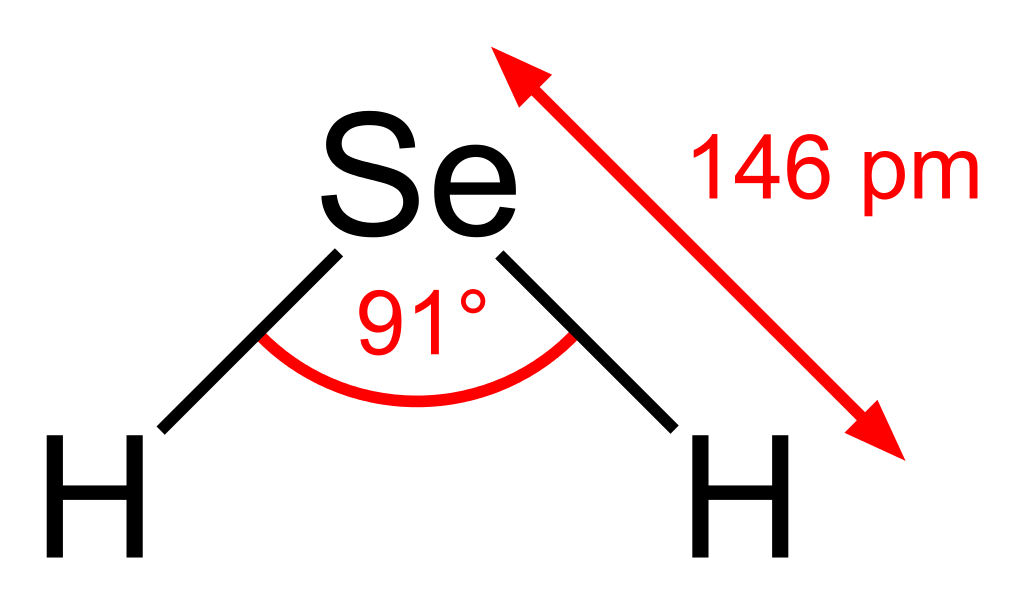# Hydrogen selenide (H2Se) lewis dot structure, molecular geometry, bond angle, polar or nonpolar

Home  > Chemistry Article > H2Se lewis structure and its molecular geometryHydrogen selenide is colorless and flammable gas with the chemical formula H2Se. It smells resemble decayed horseradish at low to medium concentrations but at higher concentrations, it smells resemble rotten eggs.

In this article, we will discuss Hydrogen selenide (H2Se) lewis structure, molecular geometry, bond angle, polar or nonpolar, etc.

Hydrogen selenide is extremely toxic and needs a lot of precaution to handle. It is the most toxic selenium compound.

 Name of Molecule Hydrogen selenide Chemical formula H2Se Molecular geometry of H2Se Bent Electron geometry of H2Se Tetrahedral Nature Slightly Polar Bond angle 91º Total Valence electron for H2Se 8
Page Contents

## How to draw lewis structure of H2Se?

H2Se lewis structure contains one selenium atom at the center position and two hydrogens on either side of it. There are a total of 2 lone pairs and 2 bond pairs present in the lewis structure H2Se.

The lewis structure of H2Se is very similar to the lewis structure of H2S, this is because both sulfur and selenium have the same valence electron since they are in the same group in the periodic table.

## Follow some steps for drawing the lewis dot structure for H2Se

1. Count total valence electron in H2Se

The first and foremost step is to determine the total number of valence electrons in H2Se. As the lewis structure is a pictorial representation of electrons around the atoms in a molecule.

Now, the hydrogen atom has only one valence electron in its outermost shell and selenium is the element of the 16th group in the periodic table, hence, it has 6 valence electrons in its outermost shell.

⇒ Total number of the valence electrons in selenium = 6

⇒ Total number of the valence electrons in hydrogen = 1

∴ Total number of valence electrons available for the H2Se Lewis structure = 6 + 1(2) = 8 valence electrons         [∴H2Se molecule has one selenium and two hydrogen atoms]2. Find the least electronegative atom and place it at center

The best preferable element for the central position is the least electronegative atom in the molecule. But it should be noted that the hydrogen atom always goes outside in the lewis diagram because it can only make a maximum of one bond.

So, in the case of H2Se, put selenium in the center and hydrogen atoms on either side of it.3. Connect outer atoms to central atom with a single bond

Now we have to make the skeletal structure of H2Se. Just place the single bonds between selenium and hydrogen atoms.Look at the above structure and determine the number of valence electrons is used till now. A single bond contains 2 electrons.

In the above structure, two single bonds are used, hence, (2 × 2) = 4 valence electrons are used in the above structure from available 8 total valence electrons.

∴ (8 – 4) = 4 valence electrons

So, we are left with 4 valence electrons more.

4. Complete the octet of the atoms

At the end, what matters for the atoms is most is that fulfilling their octet to attain stability. In the lewis diagram, the octet is filled starting from the more electronegative atoms(outer) to the less electronegative atom(central).

Now, in the case of H2Se, the hydrogen is the outer atom and it needs only 2 electrons to fulfill the octet since it has only 1s shell which can be filled maximum of 2 electrons.

The hydrogen atoms already completed their octet since they have 2 electrons(one single bond) in their valence shell.

Now, the central atom selenium needs a total of 8 electrons to complete the octet and we have 4 remaining valence electrons left as we already discussed in the 3rd step.

Let’s put these 4 remaining valence electrons over the selenium central atom.### H2Se lewis structure

That’s all, all atoms of H2Se completed their octet, as in the above structure, the central atom selenium has a total of 8 electrons(4 represented as dots + 2 single bonds that contains 4 electrons), so, it’s satisfied the octet rule.

And hydrogen has already has satisfied octet since it contains 2 electrons(one single bond) to have a full outer shell.

Now the next task of our is to calculate the formal charge for the above H2Se structure to check whether all atoms have the lowest possible formal charge..

5. Check the stability with the help of a formal charge concept

To calculate the formal charge on an atom. Use the formula given below-

⇒ Formal charge = (valence electrons – nonbonding electrons –  1/2 bonding electrons)

The nonbonding electrons are basically lone pair electrons.

Let’s calculate the formal charge for selenium and hydrogen atoms in the H2Se lewis structure.

For selenium atom:

⇒ Valence electrons of selenium = 6

⇒ Nonbonding electrons on selenium= 4

⇒ Bonding electrons around selenium(2 single bond) = 4

∴ (6 – 4 – 4/2) = 0 formal charge on selenium central atom.

For hydrogen atom

⇒ Valence electrons of hydrogen = 1

⇒ Nonbonding electrons on hydrogen = 0

⇒ Bonding electrons around hydrogen (1 single bond) = 2

∴ (1 – 0 – 2/2) = 0 formal charge on the hydrogen atom.That’s it, all the atoms have zero formal charges in the above H2Se lewis structure, hence, this is the most stable and appropriate lewis diagram of hydrogen selenide.

## What is the molecular geometry of H2Se?

The molecular geometry of H2Se is Bent because this is an AX2N2 type molecule that shows Selenium is the central atom that is attached with two bond pairs and contains 2 lone pairs, these electron pairs(lone pair and bond pair) will repel each other, this repelling effect between these electron pairs turns the bond pair linear shape to bent or V-shaped like structure.

The presence of two lone pairs on the central atom Selenium is responsible for the bent molecular shape of H2Se.

H2Se has four regions of electron density(2 bond pairs + 2 lone pairs), hence, it is an AX2N2 type molecule, according to the VSEPR chart, its molecular geometry will be bent and its electron geometry will be tetrahedral.## The bond angle of H2Se

H2Se forms an H−Se−H bond angle of 91°.## Hydrogen selenide polarity: is H2Se polar or nonpolar?

Is H2Se polar or non-polar? H2Se is very slightly polar because of its bent geometrical shape and very small difference between the electronegativity of hydrogen and selenium, although, the polarity is so low between the atoms that H2Se is also considered as nonpolar in nature.

⇒ The electronegativity of hydrogen = 2.2

⇒ The electronegativity of selenium = 2.55

∴ The difference of electronegativity between selenium and hydrogen = 0.35

According to the Pauling scale, the bond is said to be nonpolar when the difference of electronegativity between the atoms is less than 0.5.

So, in the case of H2Se, the difference in electronegativity between hydrogen and selenium is 0.35 which is less than 0.5, hence, the bond(H-Se) is nonpolar.

Since the selenium(2.55) is a little more electronegative than the hydrogen atom(2.2), the partially negative charge will induce on the selenium atom and partially positive charge on the hydrogen atom. As a result, they will create a dipole moment.

Because of this separation of charge, the dipole forms along with the bond and it will not cancel out because of the V-shaped structure of H2Se.

All this makes, H2Se slightly polar but due to the absence of enough polarity, this molecule can also be considered nonpolar in nature.

## FAQ

### How the lewis structure of H2Se is related to the H2S structure?

H2Se and H2S have a lot of common things like both contain two atoms of hydrogen, both have bent molecular structure, the central atom of H2S and H2Se is sulfur and selenium, these both belong to the same group and same family in the periodic table, both these have the same number of valence electron.

So, the available valence electrons for drawing the lewis structure of H2Se and H2S are also the same which is 8.

Both molecules (H2S and H2Se) lewis structure has 2 bonded pairs and 2 lone pairs.

### Why the molecular geometry of H2Se is bent but its electron geometry is tetrahedral?

The molecular geometry considers only the atoms of the molecule while determining the shape whereas the electron geometry considers all the electron pairs(lone pairs and bond pairs) to predict the geometry of the molecule.

Now, as per the H2Se lewis structure, the selenium central atom is attached with only two atoms and the lone pair on it will push down these bonded atoms and makes it shape like bent.

Now, the electron geometry of H2Se is tetrahedral since the selenium central atom is surrounded by 4 electron pairs(2 bond pairs + 2 lone pairs).

∴ Four regions of electron density always form a tetrahedral geometry, hence, the electron geometry of H2Se is tetrahedral.

Properties of Hydrogen selenide

• It is soluble in CS2, phosgene.
• It has a boiling point of −41.25 °C and a melting point of −65.73 °C.
• It is Extremely toxic, hazardous, dangerous, and flammable.
• The conjugate acid of H2Se is Selenonium and the conjugate base is Selenide.
• Even on small exposure, this gas can attack the eyes and mucous membranes.

Preparation of Hydrogen selenide

In the laboratory method, the hydrogen selenide is prepared by treating 6 moles of water with one mole of Aluminum selenide.

⇒ Al2Se3 + 6 H2O ⇌ 2 Al(OH)3 + 3 H2Se

In the industrial method, the hydrogen selenide is directly prepared by treating the elemental selenium with hydrogen gas at a temperature above 300ºC.

⇒ 2H + Se → H2Se

The elemental selenium can be separated from the hydrogen selenide through a reaction with sulfur dioxide.

⇒ 2 H2Se + SO2 ⇌ 2 H2O + 2 Se + S

## Summary

• The total valence electron is available for drawing the H2Se Lewis structure is 8.
• The molecular geometry of H2Se is bent because of the repelling effect caused by the presence of two lone pairs on the selenium central atom.
• The lewis structure of H2Se has 2 bonding pairs means 4 bonding electrons and 2 lone pairs means 4 nonbonding electrons.
• H2Se adopts a bent structure with an H−Se−H bond angle of 91°.
• H2Se is slightly polar, it can also be considered nonpolar because of its very low polarity due to the very low difference between the electronegativity of selenium and hydrogen atoms.
##### Subscribe to Blog via Email

Join 2 other subscribers

Share it...